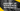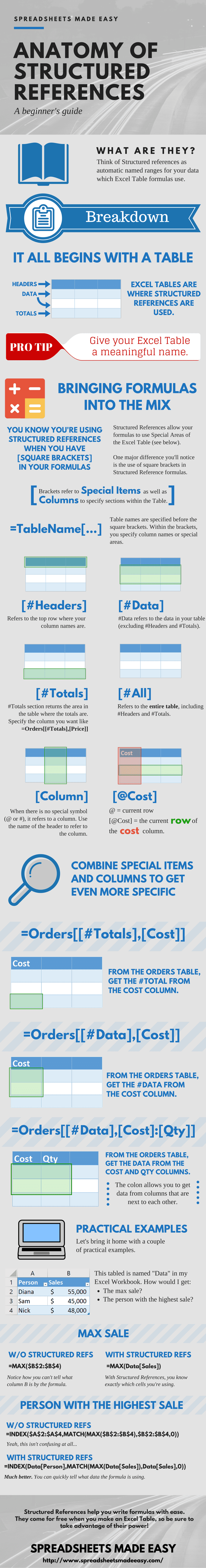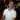# Structured References [Infographic]

June 05, 2016

Are your formulas driving you crazy? Do they have a multitude of \$ signs, colons, and letters referring to various columns? How many times have you looked at your complicated formulas and asked yourself “What data is this referring to?” I know I have. I mean, it’s not always easy remembering what \$B2:\$D10 is referring to. Luckily, we can leverage Structured References to make our formulas easier to read and write.

Check out the Infographic below. It shows your a breakdown of how Structured References work and provides some examples.## Structured References - An Interactive Example

Now that you’ve seen the Infographic, do you think Structured References will help with your formulas?

Yes, they’re obviously amazing, but how can I use them?

Great point. Let’s use an interactive example to get a better idea.

### Calculating the Total for Each Line Item

Below, there is an embedded Excel spreadsheet with an Excel Table named “Orders”.

Let’s start by calculating the total for each line item using Structured References:

1. Click in Cell D2
2. Type in the following formula: `=[@Price]*[@Quantity]`

• Remember from the Infographic that:
• The @ symbol represents the current row of the formula
• Squre Brackets [ ] are used to define special areas, like column names
3. Press Enter
4. You will see that you get the total for each line item and the Excel Table will also auto-populate the rest of the Total column.

Notice that you didn’t have to type in the Table name “Orders” in the formula. This is because you wrote the formula within the Table itself, so Excel can infer which table you are referencing.

Pretty sweet, eh?

### Getting the Total Order Amount

Let’s do one more quick example to show how to use formulas outside of the Table.

Please Note: the Table below is named Orders2. (I had to use a different table name because this is a new worksheet that we’re using)

Now that you have the Total calculated, let’s reference the total from outside of the table.

1. Click on cell B10
2. Type in the following formula: `=Orders2[[#Totals],[Total]]`

• Remember that #Totals represents the last row of the Table.
• You also have to specify which #Totals column you want, which is why you need an extra set of square brackets [ ]
3. Press Enter

Now you know how to easily reference a specific item within the Excel Table using Structured References :) awesome.

Quick question: how do you think you would get the Total Order Amount if you didn’t have Structured References?

Assuming you didn’t have a Totals row at the end of the table, it would be `=SUM(\$D:\$D)`. This may seem easy at first, but after a month or two, when you go back to your formula, you may not remember what column D has in it.

You can also reference the Total Order Amount using the SUM formula like so:

``=SUM(Orders2[Total])``

Clearly, this is much easier to read.

I hope this helps you to understand why Structured References are so powerful and how easy they are to use.